# JP2005094917A - Fuel cell system - Google Patents

## Info

Publication number
JP2005094917A
JP2005094917A JP2003324611A JP2003324611A JP2005094917A JP 2005094917 A JP2005094917 A JP 2005094917A JP 2003324611 A JP2003324611 A JP 2003324611A JP 2003324611 A JP2003324611 A JP 2003324611A JP 2005094917 A JP2005094917 A JP 2005094917A
Authority
JP
Japan
Prior art keywords
power
time
power supply
fuel cell
coefficient
Prior art date
Legal status (The legal status is an assumption and is not a legal conclusion. Google has not performed a legal analysis and makes no representation as to the accuracy of the status listed.)
Pending
Application number
JP2003324611A
Other languages
Japanese (ja)
Inventor
Toshiyuki Sugiura

Original Assignee
Nissan Motor Co Ltd

Priority date (The priority date is an assumption and is not a legal conclusion. Google has not performed a legal analysis and makes no representation as to the accuracy of the date listed.)
Filing date
Publication date
Application filed by Nissan Motor Co Ltd, 日産自動車株式会社 filed Critical Nissan Motor Co Ltd
Priority to JP2003324611A priority Critical patent/JP2005094917A/en
Publication of JP2005094917A publication Critical patent/JP2005094917A/en
Application status is Pending legal-status Critical

## Images

•••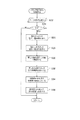•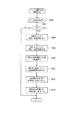•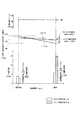•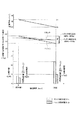•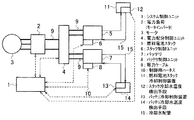•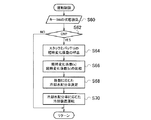•••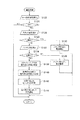•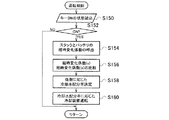•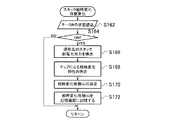•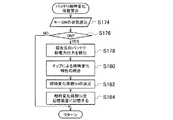•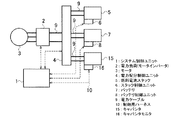## Classifications

• YGENERAL TAGGING OF NEW TECHNOLOGICAL DEVELOPMENTS; GENERAL TAGGING OF CROSS-SECTIONAL TECHNOLOGIES SPANNING OVER SEVERAL SECTIONS OF THE IPC; TECHNICAL SUBJECTS COVERED BY FORMER USPC CROSS-REFERENCE ART COLLECTIONS [XRACs] AND DIGESTS
• Y02TECHNOLOGIES OR APPLICATIONS FOR MITIGATION OR ADAPTATION AGAINST CLIMATE CHANGE
• Y02TCLIMATE CHANGE MITIGATION TECHNOLOGIES RELATED TO TRANSPORTATION
• Y02T10/00Road transport of goods or passengers
• Y02T10/60Other road transportation technologies with climate change mitigation effect
• Y02T10/72Electric energy management in electromobility
• Y02T10/7208Electric power conversion within the vehicle
• Y02T10/7241DC to AC or AC to DC power conversion

## Abstract

PROBLEM TO BE SOLVED: To reduce heat generation by reducing internal power loss in each power supply means of a fuel cell stack and at least one power storage means.
A system control apparatus (1) includes a time change coefficient indicating a degree of change over time of a fuel cell stack (5) and a change over time of a battery (7) based on state signals input from a stack control unit (6) and a battery control unit (8). Estimate the coefficient of change over time indicating the degree. Based on the estimated coefficient of change with time, the system control device 1 determines the distribution of the supplied power from the fuel cell stack 5 and the battery 7 to the motor inverter 2 that is a power load, and supplies the motor inverter 2 with the determined distribution ratio. The power distribution control unit 4 is controlled so that power is supplied.
[Selection] Figure 1

## Description

The present invention relates to a fuel cell system that supplies power to a power load from a fuel cell and power storage means, and in particular, an output of a fuel cell system capable of distributing power to the power load according to the degree of deterioration of the fuel cell and power storage means. The present invention relates to a control device.

In a fuel cell, a fuel gas such as hydrogen gas and an oxidizing gas containing oxygen are electrochemically reacted through an electrolyte, and electric energy is directly taken out between electrodes provided on both surfaces of the electrolyte. In particular, solid polymer fuel cells using solid polymer electrolytes are attracting attention as power sources for electric vehicles because of their low operating temperature and easy handling. That is, a fuel cell vehicle is equipped with a hydrogen storage device such as a high-pressure hydrogen tank, a liquid hydrogen tank, or a hydrogen storage alloy tank in the vehicle, and reacts by supplying hydrogen supplied therefrom and air containing oxygen to the fuel cell. This is the ultimate clean vehicle that drives the motor connected to the drive wheels with the electric energy extracted from the fuel cell, and the only exhaust material is water.

In a fuel cell vehicle equipped with a fuel cell and a secondary battery as power supply means, the temperature of the fuel cell stack is monitored, and when it is determined that the temperature is equal to or lower than a predetermined temperature, power generation of the fuel cell stack is limited. It is shown that the power required from the power load is covered by supplying power from the secondary battery (for example, Patent Document 1).

Further, in the output power distribution control in the fuel cell system including the conventional fuel cell and the secondary battery, the power supply means always supplies power at a constant power distribution ratio after the warm-up of the fuel cell is completed. Power was being supplied to the load.
JP 2002-034103 A (page 3, FIG. 2)

However, the fuel cell and the secondary battery differ in the degree of increase in internal resistance due to changes over time, etc. Therefore, if a constant power distribution control is continued, a specific power supply means having an increased internal resistance value There was a problem in that a large amount of heat was generated and the efficiency was reduced by this amount of heat generation.

Further, it is known that the rate at which electric power is wasted and generated as Joule heat increases as time-dependent changes progress and power transmission efficiency decreases. The heat generated due to the power loss causes the power supply means to generate more heat than necessary, and the amount of heat generated by these heat generations causes the power supply means to become high temperature, causing deterioration of the power supply means and shortening the life.

By the way, when the internal resistance value increases, the amount of heat generated per unit time increases, which also means that the temperature gradient of the power supply means becomes steep, and it can be easily imagined that the temperature variation at the heat source also increases. If the temperature variation is large, the amount of thermal strain also increases, and the influence of the thermal strain on the change over time increases, resulting in shortening the life of the power supply means.

In order to solve the above problems, the present invention provides a fuel cell system capable of supplying power to a power load from any of these power supply means, using the fuel cell stack and at least one power storage means as power supply means. A time-varying coefficient estimating means for estimating a time-varying coefficient indicating the degree of each time-varying for each power supply means, and based on the time-varying coefficient estimated by the time-varying coefficient estimating means, the power supply means for each power supplying means. The gist of the invention is that it includes power distribution control means for changing the distribution of the supplied power to the power load.

According to the present invention, since the distribution of the supply power from each power supply means to the power load can be changed according to the time-dependent change state of the plurality of power supply means, it is possible to reduce the wasteful power consumption. In addition, there is an effect that the amount of heat generated inside the power supply means can be suppressed and the change with time can be delayed.

Next, embodiments of the present invention will be described in detail with reference to the drawings. Each of the following embodiments shows a fuel cell system suitable as a power source for a fuel cell vehicle.

FIG. 1 is a configuration diagram illustrating the configuration of a first embodiment of a fuel cell system according to the present invention. 2 to 4 are control flowcharts executed by the system control unit in this embodiment, FIG. 2 is an operation control flowchart, FIG. 3 is a flowchart for calculating a coefficient of change with time of the fuel cell stack, and FIG. 4 is a change with time of the battery. It is a flowchart which calculates a coefficient.

In FIG. 1, the fuel cell system of the present embodiment is operated from electric power such as a system control unit 1 for controlling the entire system, a motor inverter 2 for controlling motor power consumption as an electric power load, and driving a vehicle. A motor 3 for extracting energy, a fuel cell stack 5 as one of power supply means, a stack control unit 6 for controlling the power generation of the fuel cell stack 5 and monitoring the state and temperature of the fuel cell stack, The battery 7 as a storage means, the battery control unit 8 for monitoring the state of charge (SOC) and temperature of the battery 7, the distribution of electric power supplied from the fuel cell stack 5 and the battery 7 to the motor inverter 2, and the motor 3 Power for charging the battery 7 with regenerative power from the battery or power generated in the fuel cell stack 5 And minute control unit 4, a power cable 9 for exchanging power, and a control harness 10 for exchanging control signals.

The system control unit 1 is not particularly limited, and is composed of, for example, a microcomputer including a program ROM, a working RAM, an input / output interface, and a CPU.

The power distribution control unit 4 is a circuit that controls power supply from the fuel cell stack 5 and the battery 7 to the motor inverter 2 that is a power load at a power distribution ratio instructed from the system control unit 1. For this reason, the power distribution control unit 4 includes, for example, as many switching circuits (two sets in this embodiment) as the number of power supply means including semiconductor switching elements such as MOS-FETs and IGBTs, and outputs of the switching circuits. An ower circuit using a diode for summing and a smoothing circuit for smoothing the output of the ower circuit are provided. Two sets of switching circuits are assigned to the output of the fuel cell stack 5 and the output of the battery 7 respectively, and the supply current from each power supply means is turned on / off at a duty according to the power distribution ratio specified by the system control unit 1. Switch. The switched supply current is summed by an ower circuit, smoothed by a smoothing circuit, and output.

The stack control unit 6 detects the state of the fuel cell stack 5 and notifies the system control unit 1 via the control harness 10 as a state signal. Examples of the status signal include the voltage, current, temperature of the fuel cell stack 5, the total amount of electric power that is an integrated value of the power extracted from the fuel cell stack 5, and the like.

The battery control unit 8 detects the state of the battery 7 and notifies the system control unit 1 via the control harness 10 as a state signal. Examples of the state signal include the voltage, current, temperature, state of charge (SOC) of the battery 7, and the total amount of electric power that is an integrated value of the electric power extracted from the battery 7.

Based on the state signals input from the stack control unit 6 and the battery control unit 8, the system control device 1 is a time change coefficient indicating the degree of change over time of the fuel cell stack 5 and the time change indicating the degree of change over time of the battery 7. Estimate the coefficient of change. Then, the system control device 1 determines the distribution of the supplied power from the fuel cell stack 5 and the battery 7 to the motor inverter 2 that is a power load based on the estimated change coefficient with time, and the motor inverter is determined with the determined distribution ratio. The power distribution control unit 4 is controlled so that power is supplied to the power source 2.

Next, the control flow in Example 1 is demonstrated with reference to FIG. 2 thru | or FIG. FIG. 2 is an operation control flow executed during normal operation, and is called and executed by the system control unit 1 at regular intervals.

In FIG. 2, first, in step (hereinafter, step is abbreviated as S) 10, the system control unit reads the state of a key switch (hereinafter, key SW). Next, in S12, it is determined whether the state of the key SW is on (ON) or off (OFF). If it is not on, the flow is simply exited and nothing is done.

If it is determined in S12 that the key SW is on, the process proceeds to S14, and the temporal change coefficient (s) of the fuel cell stack 5 and the temporal change coefficient (b) of the battery 7 are called. These time-varying coefficients are calculated by each time-varying coefficient calculating routine described later with reference to FIGS. 3 and 4 and stored in the system control unit 1.

Next, in S16, the temporal change coefficient (s) and the temporal change coefficient (b) are compared, and in S18, from the fuel cell stack 5 and the battery 7 to the motor inverter 2 that is a power load according to these temporal change coefficients. The power distribution ratio of the power to be supplied is determined. The actual power distribution amount is determined by multiplying the required power by the power distribution rate to determine the power distribution amount from the fuel cell stack 5 and the battery 7 serving as each power supply means to the motor inverter 2.

Here, a detailed example of a method for determining the power distribution rate based on the temporal change coefficient (s) and the temporal change coefficient (b) will be described.

First, let Pdst0 be the basic power distribution ratio before change with time. The power distribution rate Pdst0 indicates the power distribution rate on the battery side, and the power distribution rate on the fuel cell side is (1-Pdst0). In FIGS. 5 and 6 described later, Pdst = 0.6 is called the battery auxiliary rate 60 [%].

Then, the corrected battery power distribution rate Pdst considering the time variation coefficient is expressed by the following formula (1) using the basic power distribution rate Pdst0, the time variation coefficient (s), and the time variation coefficient (b). Can be calculated.

(Equation 1)
Pdst = Pdst0 × {1 + k (time-varying coefficient (b) −time-varying coefficient (s))} (1)
Here, k is a coefficient that can be arbitrarily set according to the characteristics of the system.
At this time, the corrected power distribution ratio of the fuel cell stack using Pdst is (1-Pdst).

Finally, in S20, the power distribution control unit is controlled to control the power supplied from the fuel cell stack 5 and the battery 7 to the motor inverter 2 at the power distribution rate, and the process returns to the main routine.

Next, the calculation method of the time variation coefficient (s) and the time variation coefficient (b) in this embodiment and the method for determining the power distribution rate based on the time variation coefficient (s) and the time variation coefficient (b) will be described in detail. To do.

The time-varying coefficient (s) of the fuel cell stack stores the voltage current characteristics of the current or the latest fuel cell stack, and the design voltage current characteristics stored in advance or the voltage current characteristics at the time of manufacturing the fuel cell system are used as reference values. The current characteristic value can be obtained by dividing by the reference value.

More specifically, the output voltage value V0 of the fuel cell stack at a predetermined output current value I0 or the output voltage values V1, V2,..., Vn at several output current values I1, I2,. . These output voltage values V0, V1, V2,..., Vn decrease with time. Therefore, the detection value V0 is divided by the reference value VS0 stored in advance, or the detection values V1, V2,..., Vn are divided by the reference values VS1, VS2,. Thus, the stack aging coefficient (s) is set as shown in the following formula (2) or formula (3). The time variation coefficient (s) indicates that the smaller the numerical value, the lower the output capability due to the time variation.

(Equation 2)
Coefficient of change with time (s) = V0 / VS0 (2)
Coefficient of change with time (s) = (1 / n) (V1 / VS1 + V2 / VS2 + ... + Vn / VSn) (3)
Similarly, the battery aging coefficient (b) is the output voltage value v0 of the battery at a predetermined output current value i0 or the output voltage values v1, v2,..., At several output current values i1, i2,. Detect vn. These output voltage values v0, v1, v2,..., Vn decrease with time. Therefore, the detection value v0 is divided by the reference value vS0 stored in advance, or the detection values v1, v2,..., Vn are divided by the reference values vS1, vS2,. Thus, the time-dependent change coefficient (b) of the battery is defined as in the following formula (4) or formula (5). This time-dependent change coefficient (b) indicates that the smaller the numerical value, the lower the output capability due to time-dependent change.

(Equation 3)
Coefficient of change with time (b) = v0 / vS0 (4)
Coefficient of change with time (b) = (1 / n) (v1 / vS1 + v2 / vS2 + ... + vn / vSn) (5)
Next, the stack aging coefficient (s) calculation process will be described with reference to FIG.
In FIG. 3, first, in S22, the system control unit reads the state of the key SW. Next, in S24, it is determined whether the state of the key SW is on (ON) or off (OFF). If it is not on, the flow is simply exited and nothing is done.

If it is determined in S24 that the key SW is on, the process proceeds to S26, and the current voltage-current characteristic of the fuel cell stack 5 is detected. Next, in S28, the voltage-current characteristics of the designed fuel cell stack stored in advance are called, and in S30, the current value is divided by the designed value. Next, in S32, the value obtained in S30 is defined as the stack design aging rate, and in S34, the stack design aging rate is defined as the stack aging coefficient (s). In step S36, the coefficient of change with time (s) is stored in the storage device inside the system control unit, and the process returns.

Next, with reference to FIG. 4, the battery aging coefficient (b) calculation process will be described.
In FIG. 4, first, in S40, the system control unit reads the state of the key SW. Next, in S42, it is determined whether the state of the key SW is on (ON) or off (OFF). If it is not on, the flow is simply exited and nothing is done.

If it is determined in S42 that the key SW is on, the process proceeds to S44, and the current voltage-current characteristic of the battery 7 is detected. Next, in S46, the voltage / current characteristic of the designed battery stored in advance is called, and in S48, the current value is divided by the designed value. Next, in S50, the value obtained in S48 is defined as the battery design aging rate, and in S52, the battery design aging rate is defined as the battery aging coefficient (b). In S54, the coefficient of change with time (b) is stored in the storage device inside the system control unit, and the process returns.

If the key SW remains in the ON state, the flow of FIG. 2 is repeated again to call up the temporal change coefficient of the fuel cell stack or battery temporarily stored, and the power distribution correction of each power supply means is performed based on this temporal change coefficient. Do.

In addition to the voltage-current characteristics, an internal resistance value is calculated based on the voltage-current characteristics of each power supply means as a time-varying coefficient indicating the degree of time-dependent change of each power supply means, and an initial value ( The rate of change with respect to the design value) may be a change coefficient with time. Alternatively, the open-circuit voltage of each power supply unit may be detected, and an initial value (design value) of the open-circuit voltage may be calculated based on a change in the internal resistance value of each power supply unit. Furthermore, the minimum voltage or average voltage of each cell or each module constituting each power supply means may be detected, and the rate of change of these voltages with respect to the initial value (design value) may be used as the time variation coefficient.

FIG. 5 shows the power loss of the comparative example, and FIG. 6 is a diagram showing the power loss of this example. In any case, the effective power ratio τ at which the generated power in the fuel cell stack can be output is the design value (initial value) 90 [%], the current value after change with time is 85 [%], and the output power relative to the input power of the battery The ratio τ is a design value (initial value) 95 [%], and the current value after change with time is 75 [%].

In the comparative example of FIG. 5, since the battery auxiliary rate of power supplied to the power load is fixed to 60 [%], the power loss at the design value (initial value) is 4 [kW] for the fuel cell stack. The battery capacity is 3 [kW], and the total power loss is 7 [kW]. At the present time after a change over time and operation for a predetermined time, the power loss is 6 [kW] for the fuel cell stack, 15 [kW] for the battery, and the total power loss is 21 [kW].

On the other hand, in the present embodiment of FIG. 6, since the power distribution to the power load is changed according to the time variation coefficient, the battery auxiliary rate changes with time from the design value (initial value) 60 [%]. It decreases to 20 [%] along with. For this reason, the power loss at the design value (initial value) is 4 [kW] for the fuel cell stack and 3 [kW] for the battery, and the total power loss is 7 [kW] and does not change. However, at the present after a predetermined time operation due to the progress of change over time, the power loss is 12 [kW] for the fuel cell stack, 5 [kW] for the battery, and 17 [kW] for the total power loss. On the other hand, in the embodiment, the total power loss is reduced by 4 [kW].

As described above, according to the present embodiment, it is possible to suppress power loss that is changed to heat inside the power supply means by performing power distribution correction from each power supply means to the power load based on the coefficient of change over time. it can.

Further, since the amount of generated heat is reduced, the temperature increase of the power supply means is suppressed, and the change with time of the power supply means can be suppressed. The power reduced by the power distribution correction is covered by the power supply means having a relatively small change coefficient with time, and is made to correspond to the demand for the power load.

FIG. 7 is a configuration diagram illustrating the configuration of a second embodiment of the fuel cell system according to the present invention. FIGS. 8 to 10 are control flowcharts executed by the system control unit according to the present embodiment, FIG. 8 is an operation control flowchart, FIG. 9 is a flowchart for calculating a time-dependent change coefficient (s) of the fuel cell stack, and FIG. It is a flowchart which calculates a time-dependent change coefficient (b).

7, the fuel cell system of the present embodiment is different from the system of the first embodiment of FIG. 1 in that the fuel cell stack cooling control device 11 has a variable cooling capacity for cooling the fuel cell stack 5, and the fuel cell stack. 5, a cooling water temperature detecting means 12 for detecting the cooling water temperature, a battery cooling control device 13 having a variable cooling capacity for cooling the battery 7, and a battery cooling water temperature detecting means for detecting the cooling water temperature of the battery 7. 14 and a cooling water pipe 15 are added. Since the other configuration is the same as that of the first embodiment shown in FIG. 1, the same components are assigned the same reference numerals, and redundant description is omitted.

Although not shown, the fuel cell stack cooling control device 11 and the battery cooling control device 13 may be configured so that the cooling capacity can be changed independently of each other, but in this embodiment, the fuel cell stack cooling control is performed. The device 11 and the battery cooling control device 13 share a radiator that radiates heat of the cooling water, a cooling water pump, and the like, and change the distribution ratio of the cooling water to the fuel cell stack cooling control device 11 and the battery cooling control device 13. Therefore, the cooling capacity is changed.

Next, a control flow in the second embodiment will be described with reference to FIGS. FIG. 8 is an operation control flow executed during normal operation, and is called and executed by the system control unit 1 at regular intervals.

In FIG. 8, first, in S60, the system control unit reads the state of the key SW. Next, in S62, it is determined whether the state of the key SW is on (ON) or off (OFF). If it is not on, the flow is simply exited and nothing is done.

If it is determined in S62 that the key SW is on, the process proceeds to S64, and the temporal change coefficient (s) of the fuel cell stack 5 and the temporal change coefficient (b) of the battery 7 are called. These time-varying coefficients are calculated by each time-varying coefficient calculating routine described later with reference to FIGS. 3 and 4 and stored in the system control unit 1.

Next, in S66, the temporal change coefficient (s) and the temporal change coefficient (b) are compared, and in S68, the distribution ratio of the cooling water supplied to the fuel cell stack 5 and the battery 7 is determined in accordance with these temporal change coefficients. decide.

Finally, in S70, the cooling water is supplied to the fuel cell stack 5 and the battery 7 at this cooling water distribution ratio, the fuel cell stack cooling control device 11 and the battery cooling control device 13 are operated, and the process returns to the main routine.

Next, the stack aging coefficient (s) calculation process will be described with reference to FIG.
In FIG. 9, first, in S72, the system control unit reads the state of the key SW. Next, in S74, it is determined whether the state of the key SW is on (ON) or off (OFF). If it is not on, the flow is simply exited and nothing is done.

If it is determined in S74 that the key SW is on, the process proceeds to S76, and the current power-temperature characteristic of the fuel cell stack 5 is detected. This power-temperature characteristic is obtained as the temperature of the fuel cell stack 5 detected by the stack cooling water temperature detection means 12 with respect to the output power of the fuel cell stack 5.

Next, in S78, the power-temperature characteristic of the designed fuel cell stack stored in advance is called, and in S80, the current value is divided by the designed value. Next, in S82, the value obtained in S80 is defined as the stack design aging rate, and in S84, the stack design aging rate is defined as the stack aging coefficient (s). In S86, the coefficient of change with time (s) is stored in the storage device inside the system control unit.

Next, in S88, it is determined whether or not this routine is executed for the first time since the start of the fuel cell. If it is not the first time after startup, this routine is terminated and the process returns to the main routine. If it is determined in S88 that this is the first time after startup, the process proceeds to S90, where power-temperature characteristics, which are temperature characteristics with respect to the output power of the fuel cell stack 5 immediately after startup, are detected, and in S92, power-temperature characteristics immediately after startup. Is stored in the storage device inside the system control unit, and the process returns to the main routine.

Next, the battery aging coefficient (b) calculation process will be described with reference to FIG.
In FIG. 10, first, in S100, the system control unit reads the state of the key SW. Next, in S102, it is determined whether the state of the key SW is on (ON) or off (OFF). If it is not on, the flow is simply exited and nothing is done.

If it is determined in S102 that the key SW is on, the process proceeds to S104, and the current power-temperature characteristic of the battery 7 is detected. This power-temperature characteristic is obtained as the temperature of the battery 7 detected by the battery coolant temperature detection means 14 with respect to the output power of the battery 7.

Next, in step S106, the power-temperature characteristic of the designed battery stored in advance is called, and in S108, the current value is divided by the designed value. Next, in S110, the value obtained in S108 is defined as the battery design aging rate, and in S112, the battery design aging rate is defined as the battery aging coefficient (b). In S114, the coefficient of change with time (b) is stored in the storage device inside the system control unit.

Next, in S116, it is determined whether or not this routine is executed for the first time since the start of the fuel cell. If it is not the first time after startup, this routine is terminated and the process returns to the main routine. If it is determined in S116 that it is the first time after startup, the process proceeds to S118, where the power-temperature characteristic, which is the temperature characteristic with respect to the output power of the battery 7 immediately after startup, is detected. The data is stored in the storage device inside the control unit, and the process returns to the main routine.

According to the second embodiment described above, the fuel cell stack and the battery, which are power supply means, are each provided with a cooling means having variable cooling capacity, and the cooling capacity of the cooling means of the power supply means having a large change with time change coefficient. Since it is made to increase, it becomes possible to suppress the temperature rise of the power supply means that has changed with time and to delay the progress of the change with time of the power supply means.

The configuration of the present embodiment is the same as that of the second embodiment shown in FIG. FIG. 11 is an operation control flow executed during normal operation, and is called and executed by the system control unit 1 at regular intervals. In this embodiment, the temperature of the fuel cell stack and the battery, which are power supply means, is detected by the respective temperature detection means, and if the detected temperature reaches the upper limit temperature of each power supply means, It is characterized in that the extraction current is limited to be further reduced.

In FIG. 11, first, in S122, the system control unit reads the state of the key SW. Next, in S124, it is determined whether the state of the key SW is on (ON) or off (OFF). If it is not on, the flow is simply exited and nothing is done.

If it is determined in S124 that the key SW is on, the process proceeds to S126, and the temperature of the fuel cell stack 5 is read from the stack cooling water temperature detecting means 12. Next, in S128, it is determined whether or not the temperature has reached a predetermined upper limit temperature. If the temperature is equal to or higher than the predetermined upper limit temperature, the process proceeds to S130, and the current taken out from the fuel cell stack 5 is further limited to be smaller. End the routine and return to the main routine.

If it is determined in S128 that the predetermined upper limit temperature has not been reached, the process proceeds to S132, and the temperature of the battery 7 is read from the battery coolant temperature detecting means 14. Next, in S134, it is determined whether or not the temperature has reached a predetermined upper limit temperature. If the temperature is equal to or higher than the predetermined upper limit temperature, the process proceeds to S136, and the current taken out from the battery 7 is further limited to be smaller. End and return to the main routine.

If it is determined in S134 that the predetermined upper limit temperature has not been reached, the process proceeds to S138, and the temporal change coefficient (s) of the fuel cell stack 5 and the temporal change coefficient (b) of the battery 7 are called. These time-varying coefficients are calculated by the time-varying coefficient calculating routines of FIGS. 9 and 10 of the second embodiment and stored in the system control unit 1.

Next, in S140, the temporal change coefficient (s) and the temporal change coefficient (b) are compared, and in S142, from the fuel cell stack 5 and the battery 7 to the motor inverter 2, which is a power load, according to these temporal change coefficients. The power distribution ratio of the power to be supplied is determined.

A detailed example of the method for determining the power distribution rate based on the temporal change coefficient (s) and the temporal change coefficient (b) is as described in the first embodiment.

Finally, in S144, the power distribution control unit is controlled to control the power supplied from the fuel cell stack 5 and the battery 7 to the motor inverter 2 at the power distribution rate, and the process returns to the main routine.

In this embodiment, by correcting the power distribution according to the coefficient of change with time from the characteristics of power and temperature, the amount of output current extracted from the power supply means with increased output resistance is limited, and the power that changes to heat is suppressed. Can do. At that time, when the temperature of the power supply means detected by the temperature detection means approaches the upper limit of operation temperature, the maximum extraction current from the power supply means is reduced,
It becomes possible to protect the power supply means approaching the operation upper limit temperature and to suppress the progress of the change with time.

The configuration of the present embodiment is the same as that of the second embodiment shown in FIG. FIG. 12 is an operation control flow executed during normal operation, and is called and executed by the system control unit 1 at regular intervals. FIG. 13 is a flowchart for calculating the time variation coefficient of the fuel cell stack, and FIG. 14 is a flowchart for calculating the time variation coefficient of the battery.

The feature of the present embodiment is that the amount of power extracted from each power supply means is integrated to calculate the total power amount, and the change with time of each power supply means proceeds according to this total power amount. For this reason, on the basis of the total amount of power accumulated for each power supply means, a time-varying coefficient is obtained with reference to a previously stored control map, and each power supply means is determined by the cooling water distribution rate according to the time-varying coefficient. The cooling control device is operating. In this control map, numerical values obtained in advance by experiments are mapped and stored in the system control unit.

In FIG. 12, first, in S150, the system control unit reads the state of the key SW. Next, in S152, it is determined whether the state of the key SW is on (ON) or off (OFF). If it is not on, the flow is simply exited and nothing is done.

If it is determined in S152 that the key SW is on, the process proceeds to S154, and the temporal change coefficient (s) of the fuel cell stack 5 and the temporal change coefficient (b) of the battery 7 are called. These time-varying coefficients are calculated by each time-varying coefficient calculating routine described later with reference to FIGS. 3 and 4 and stored in the system control unit 1.

Next, in S156, the coefficient of change with time (s) and the coefficient of change with time (b) are compared. In S158, the distribution ratio of the cooling water supplied to the fuel cell stack 5 and the battery 7 is determined in accordance with these coefficients of change with time. decide.

Finally, in S160, the cooling water is supplied to the fuel cell stack 5 and the battery 7 at this cooling water distribution ratio, the fuel cell stack cooling control device 11 and the battery cooling control device 13 are operated, and the process returns to the main routine.

Next, the stack aging coefficient (s) calculation process will be described with reference to FIG.
In FIG. 13, first, in S162, the system control unit reads the state of the key SW. Next, in S164, it is determined whether the state of the key SW is on (ON) or off (OFF). If it is not on, the flow is simply exited and nothing is done.

If it is determined in S164 that the key SW is on, the process proceeds to S166, and the total amount of power extracted from the fuel cell stack 5 up to the present is detected. Next, in S168, the control map stored in advance in the system control unit is called to search the control map by the total power amount, and in S170, the time-varying coefficient corresponding to the total power amount is read from the control map, so that Change coefficient (s). Next, in S172, the value obtained in S170 is stored in the storage device inside the system control unit as the time variation coefficient (s), and the process returns.

Next, with reference to FIG. 14, the battery aging coefficient (b) calculation process will be described.
In FIG. 14, first, in S174, the system control unit reads the state of the key SW. Next, in S176, it is determined whether the state of the key SW is on (ON) or off (OFF). If it is not on, the flow is simply exited and nothing is done.

If it is determined in S176 that the key SW is on, the process proceeds to S178, and the total amount of power extracted from the battery 7 up to now is detected. Next, in S180, the control map stored in the system control unit in advance is called to search the control map by the total power amount, and in S182, the time variation coefficient corresponding to the total power amount is read from the control map to Change coefficient (b). Next, in S184, the value obtained in S182 is stored in the storage device inside the system control unit as the time variation coefficient (b), and the process returns.

If the key SW remains in the ON state, the flow of FIG. 12 is repeated again, and the temporally stored coefficient of the fuel cell stack or battery is called, and the cooling water distribution is distributed to each power supply means based on this temporally variable coefficient. Go and cool.

According to the present embodiment, the total power amount that is an integrated value of the electric power extracted from each power supply means is used as a time change coefficient, and the cooling capacity of the cooling device that cools each power supply means according to the time change coefficient is determined. It is possible to increase the temperature and suppress the temperature rise of the power supply means that has changed over time, and to delay the progress of the time change of the power supply means.

Next, a fifth embodiment of the fuel cell system according to the present invention will be described. The system configuration of the fifth embodiment is the same as that of the first embodiment shown in FIG. The same applies to the calculation method of the coefficient of change with time of the fuel cell stack and the battery as the power supply means, and the power distribution based on the number of changes with time.

In this embodiment, when the time variation coefficient of any power supply means reaches a predetermined limit or exceeds the predetermined limit, heat generation due to power loss in this power supply means becomes remarkably large, and the operation is continued as it is. If there is a problem, the power supply from this power supply means is stopped. And it becomes possible to continue a minimum driving | operation by supplying electric power to an electric power load from the remaining electric power supply means.

For this reason, the system control unit 1 receives state signals from the stack control unit 6 and the battery control unit 8 and calculates temporal change coefficients of the fuel cell stack 5 and the battery 7 based on these state signals. When the time-varying coefficient reaches a predetermined value stored in advance, the system control unit 1 stops power supply from the power supply means, assuming that the power supply means has reached the limit of change over time.

Stopping the power supply from the power supply means to the load is achieved by the power distribution control unit 4 setting the power distribution rate of the target power supply means to 0 in accordance with an instruction from the system control unit 1.

According to the embodiment described above, when the coefficient of change with time reaches a predetermined limit or exceeds the predetermined limit, the power supply from the power supply means is stopped to stop the heat generation of the power supply means. The operation can be continued with the system function reduced.

FIG. 15 is a configuration diagram illustrating the configuration of a sixth embodiment of the fuel cell system according to the present invention. In this embodiment, a battery and a capacitor are provided as power storage means.

The difference from the first embodiment shown in FIG. 1 is that a capacitor 15 and a capacitor monitor 16 for controlling charge / discharge of the capacitor 16 and monitoring the amount of charge (SOC) of the capacitor 16 are added. Is to control the distribution of the power supplied from the three power supply means of the fuel cell stack 5, the battery 7 and the capacitor 15 to the motor inverter 2 which is a power load. In the present embodiment, since the number of power supply means is 3, the power distribution control unit 4 includes three sets of switching circuits, a three-input ower circuit, and a smoothing circuit. Then, the power distribution control unit 4 switches the supply current from each power supply unit with a complementary duty according to the power distribution ratio instructed from the system control unit 1.

The method of detecting or calculating the coefficient of change with time of each power supply means can be detected or calculated by the same method as described in the above embodiments. The control flow in normal operation is the same as that in each of the above embodiments, but the power distribution rate of each power supply means is controlled based on the time variation coefficient of the three types of power supply means to reduce power loss. .

In the sixth embodiment, in particular, even when the fuel cell stack is not generating electric power and the operation using only the battery and the capacitor, which are the remaining power supply means, the respective time-varying states of the battery and the capacitor are detected. By allocating power according to the coefficient of change over time, the amount of current taken out from the power supply means with increased output resistance is limited, and the heat generation of this power supply means is effectively suppressed and the progress of deterioration is suppressed. There is an effect that it can be suppressed.

1 is a system configuration diagram illustrating the configuration of a first embodiment of a fuel cell system according to the present invention. FIG. 3 is a flowchart for explaining an operation control operation in the first embodiment. 6 is a flowchart for explaining a time-dependent change coefficient calculation operation of the fuel cell stack according to the first embodiment. 3 is a flowchart illustrating a battery temporal change coefficient calculation operation according to the first embodiment. It is a figure explaining the time-dependent change of the power loss with respect to the operation time in a comparative example. It is a figure explaining the time-dependent change of the power loss with respect to the operation time in Example 1. FIG. It is a system block diagram explaining the structure of Example 2 of the fuel cell system which concerns on this invention. 6 is a flowchart for explaining an operation control operation in the second embodiment. 6 is a flowchart illustrating a time-dependent change coefficient calculation operation of a fuel cell stack according to a second embodiment. 6 is a flowchart illustrating a battery temporal change coefficient calculation operation according to the second embodiment. 12 is a flowchart for explaining an operation control operation in the third embodiment. 10 is a flowchart illustrating an operation control operation in Embodiment 4. 10 is a flowchart illustrating a time-dependent change coefficient calculation operation of a fuel cell stack according to a fourth embodiment. 12 is a flowchart for explaining a battery temporal change coefficient calculation operation according to the fourth embodiment. It is a system block diagram explaining the structure of Example 6 of the fuel cell system based on this invention.

Explanation of symbols

1 ... System control unit 2 ... Power load (motor inverter)
DESCRIPTION OF SYMBOLS 3 ... Motor 4 ... Electric power distribution control unit 5 ... Fuel cell stack 6 ... Stack control unit 7 ... Battery 8 ... Battery control unit 9 ... Electric power cable 10 ... Control harness

## Claims (11)

1. In a fuel cell system capable of supplying power to a power load from any of these power supply means using a fuel cell stack and at least one power storage means as power supply means,
A time-varying coefficient estimating means for estimating a time-varying coefficient indicating the degree of time-dependent change for each power supply means;
Power distribution control means for determining distribution of power supplied to the power load for each power supply means based on the time change coefficient estimated by the time change coefficient estimation means;
A fuel cell system comprising:
2. The power distribution control means includes
Basic distribution amount calculating means for calculating a basic power distribution amount of each power supply means for the required supply power from the power load;
Power distribution amount correction means for correcting the basic power distribution amount based on a coefficient of change with time of each power supply means,
2. The fuel cell system according to claim 1, wherein the power distribution amount correction means reduces the power distribution amount from the power supply means having a large change in the change coefficient with time.
3. A cooling device having a variable cooling capacity for each power supply means;
3. The fuel cell system according to claim 1, wherein the cooling capacity of the cooling device of the power supply means with a large change in the coefficient of change with time is increased.
4. Temperature detecting means for detecting the temperature of each power supply means;
4. The fuel cell system according to claim 3, wherein the power distribution amount correcting means decreases the power distribution amount of the power supply means when the temperature of the power supply means becomes equal to or higher than an upper limit temperature.
5. The time-varying coefficient estimating means includes
A temperature change rate per work rate is calculated based on the temperature of each power supply means and the work rate that is the transmission power of each power supply means, and the temperature change rate per work rate is estimated as a time change coefficient. The fuel cell system according to claim 1 or 2.
6. The time-varying coefficient estimating means includes
3. The fuel cell system according to claim 1, wherein the time-varying coefficient is estimated based on an output resistance value obtained from a voltage and current characteristic of each power supply means.
7. The time-varying coefficient estimating means includes
The fuel cell system according to claim 1 or 2, wherein an open circuit voltage of each power supply means is divided by an initial open circuit voltage to obtain the time variation coefficient.
8. The time-varying coefficient estimating means includes
3. The fuel cell system according to claim 1, wherein the time-varying coefficient is obtained by dividing a minimum value or an average value of a voltage for each basic unit constituting each power supply unit by a respective initial voltage. .
9. The time-varying coefficient estimating means includes
3. The fuel cell system according to claim 1, wherein the time-varying coefficient of each power supply means is estimated based on a total power amount that is an integrated value of the power extracted from each power supply means. .
10.   3. The fuel according to claim 1, wherein when the coefficient of change with time of the power supply means reaches a predetermined limit or exceeds a predetermined limit, the power supply from the power supply means to the load is stopped. Battery system.
11. Two or more types of power storage means as the power supply means,
The power distribution control means, when not supplying power from the fuel cell stack to the power load, determines the distribution of the supplied power to the power load according to a coefficient of change with time of each power storage means. The fuel cell system according to claim 1 or 2.
JP2003324611A 2003-09-17 2003-09-17 Fuel cell system Pending JP2005094917A (en)

## Priority Applications (1)

Application Number Priority Date Filing Date Title
JP2003324611A JP2005094917A (en) 2003-09-17 2003-09-17 Fuel cell system

## Applications Claiming Priority (1)

Application Number Priority Date Filing Date Title
JP2003324611A JP2005094917A (en) 2003-09-17 2003-09-17 Fuel cell system

## Publications (1)

Publication Number Publication Date
JP2005094917A true JP2005094917A (en) 2005-04-07

# Family

## Family Applications (1)

Application Number Title Priority Date Filing Date
JP2003324611A Pending JP2005094917A (en) 2003-09-17 2003-09-17 Fuel cell system

## Country Status (1)

JP (1) JP2005094917A (en)

## Cited By (9)

* Cited by examiner, † Cited by third party
Publication number Priority date Publication date Assignee Title
JP2006048943A (en) * 2004-07-30 2006-02-16 Toyota Motor Corp Fuel cell system
JP2007282315A (en) * 2006-04-03 2007-10-25 Nissan Motor Co Ltd Fan control device of fuel battery vehicle
WO2008004464A1 (en) * 2006-07-03 2008-01-10 Toyota Jidosha Kabushiki Kaisha Voltage converter and vehicle having the same
WO2008007627A1 (en) * 2006-07-10 2008-01-17 Toyota Jidosha Kabushiki Kaisha Power supply system, vehicle using the same, and its control method
JP2008226674A (en) * 2007-03-13 2008-09-25 Honda Motor Co Ltd Fuel cell system
WO2011056998A2 (en) * 2009-11-05 2011-05-12 Ise Corporation Propulsion energy storage control system and method of control
US8004109B2 (en) 2007-01-04 2011-08-23 Toyota Jidosha Kabushiki Kaisha Vehicle power supply apparatus, and vehicle
CN101595008B (en) * 2006-12-19 2012-05-23 丰田自动车株式会社 Power control device and vehicle using the same
RU2521589C1 (en) * 2010-04-01 2014-06-27 Дае-Кё ЛИ Device and method for power compensation in vehicle by means of capacitor with high capacitance

## Cited By (12)

* Cited by examiner, † Cited by third party
Publication number Priority date Publication date Assignee Title
JP2006048943A (en) * 2004-07-30 2006-02-16 Toyota Motor Corp Fuel cell system
JP2007282315A (en) * 2006-04-03 2007-10-25 Nissan Motor Co Ltd Fan control device of fuel battery vehicle
JP4706540B2 (en) * 2006-04-03 2011-06-22 日産自動車株式会社 Fan control device for fuel cell vehicle
WO2008004464A1 (en) * 2006-07-03 2008-01-10 Toyota Jidosha Kabushiki Kaisha Voltage converter and vehicle having the same
WO2008007627A1 (en) * 2006-07-10 2008-01-17 Toyota Jidosha Kabushiki Kaisha Power supply system, vehicle using the same, and its control method
US7928603B2 (en) 2006-07-10 2011-04-19 Toyota Jidosha Kabushiki Kaisha Power supply system, vehicle using the same and its control method
CN101595008B (en) * 2006-12-19 2012-05-23 丰田自动车株式会社 Power control device and vehicle using the same
US8004109B2 (en) 2007-01-04 2011-08-23 Toyota Jidosha Kabushiki Kaisha Vehicle power supply apparatus, and vehicle
JP2008226674A (en) * 2007-03-13 2008-09-25 Honda Motor Co Ltd Fuel cell system
WO2011056998A2 (en) * 2009-11-05 2011-05-12 Ise Corporation Propulsion energy storage control system and method of control
WO2011056998A3 (en) * 2009-11-05 2011-10-13 Ise Corporation Propulsion energy storage control system and method of control
RU2521589C1 (en) * 2010-04-01 2014-06-27 Дае-Кё ЛИ Device and method for power compensation in vehicle by means of capacitor with high capacitance

## Similar Documents

Publication Publication Date Title
JP5862631B2 (en) Power storage system
KR101304729B1 (en) Voltage equalization device, method, computer-readable storage medium recording program, and power accumulation system
JP5488169B2 (en) Power supply
US9166418B2 (en) Battery system and control method thereof
US9231425B2 (en) Battery pack system of improving operating performance using internal resistance of cell
KR100685214B1 (en) Fuel cell system and control method
JP4222337B2 (en) Power supply system having a plurality of power supplies and vehicle having the same
JP4564934B2 (en) Secondary battery module
KR20160007826A (en) Driving control method of fuel cell system
JP3961950B2 (en) Hybrid power supply
JP3415740B2 (en) Battery charger
KR100835331B1 (en) Hybrid fuel cell system and voltage conversion control method thereof
EP2178148B1 (en) Fuel cell system and its control method
EP2685597A1 (en) Charge control device for vehicle
US7447572B2 (en) Control apparatus for vehicle having fuel cell mounted thereon
JP4893127B2 (en) Control device for fuel cell vehicle
EP2685598B1 (en) Charge control device for vehicle
US7710067B2 (en) Power supply system and power supply system control method
DE112009000598B4 (en) Power supply control method
JP4367251B2 (en) Power supply device and electronic device
CN100534828C (en) Fuel cell system and electric vehicle including the fuel cell system
JPWO2010125878A1 (en) Control apparatus and control method
US7629770B2 (en) Device and method for controlling output from a rechargeable battery
JP4873260B2 (en) Fuel cell system
US7771864B2 (en) Method of detecting and responding to a cooling system failure in a power supply device